Recurrent Neural Network GrammarsRNNG在语言模型任务上取得了当时的state-of-the-art结果，成分句法分析任务上，生成模型取得了媲美最好结果的F1值，而判别模型就差了点。本文最大的贡献点就是提出了生成式模型RNNG，说明了在数据量不是很大的时候，利用生成式模型可以提高成分句法分析的准确率。

# 摘要

RNN在语言模型和其他许多NLP任务上面都已经取得了非常不错的效果，但是RNN只能捕捉到句子的序列特征，例如句子的句法结构等递归嵌套的结构信息无法用RNN捕捉到。

RNNG建立在top-down转移系统之上，top-down转移系统相比于bottom-up转移系统有一个好处，就是不需要二叉化，因为如果bottom-up转移系统不二叉化的话，REDUCE的状态就会有很多种可能，不知道到底归约栈里的几个结点。而top-down转移系统就不存在这个问题，直接归约到第一个父结点为止就行了。本文应该也是第一个提出用RNN来实现top-down转移系统的，之前的方法都是用top-down的文法，或者是bottom-up的，例如Sochar2013的CVG，也是用二叉化后的RNN学习结点的语义表示。

# RNN文法

RNNG定义为三元组$(N, \Sigma, \Theta)$，其中$N$是非终结符集合，$\Sigma$是终结符集合，并且$N \cap \Sigma = \emptyset$，$\Theta$就是神经网络的参数集合。RNNG和传统的PCFG的一个明显区别就是它没有显式地指出语法规则是什么，而是蕴含在了神经网络中，在句法转移的时候动态的生成。

# Top-down句法分析和生成

## 判别式系统

• NT(X)： 将一个父结点X移进栈里。
• SHIFT： 从buffer中移一个单词到栈里。
• REDUCE： 将栈顶的若干个结点归约为它们的父结点，并且出栈。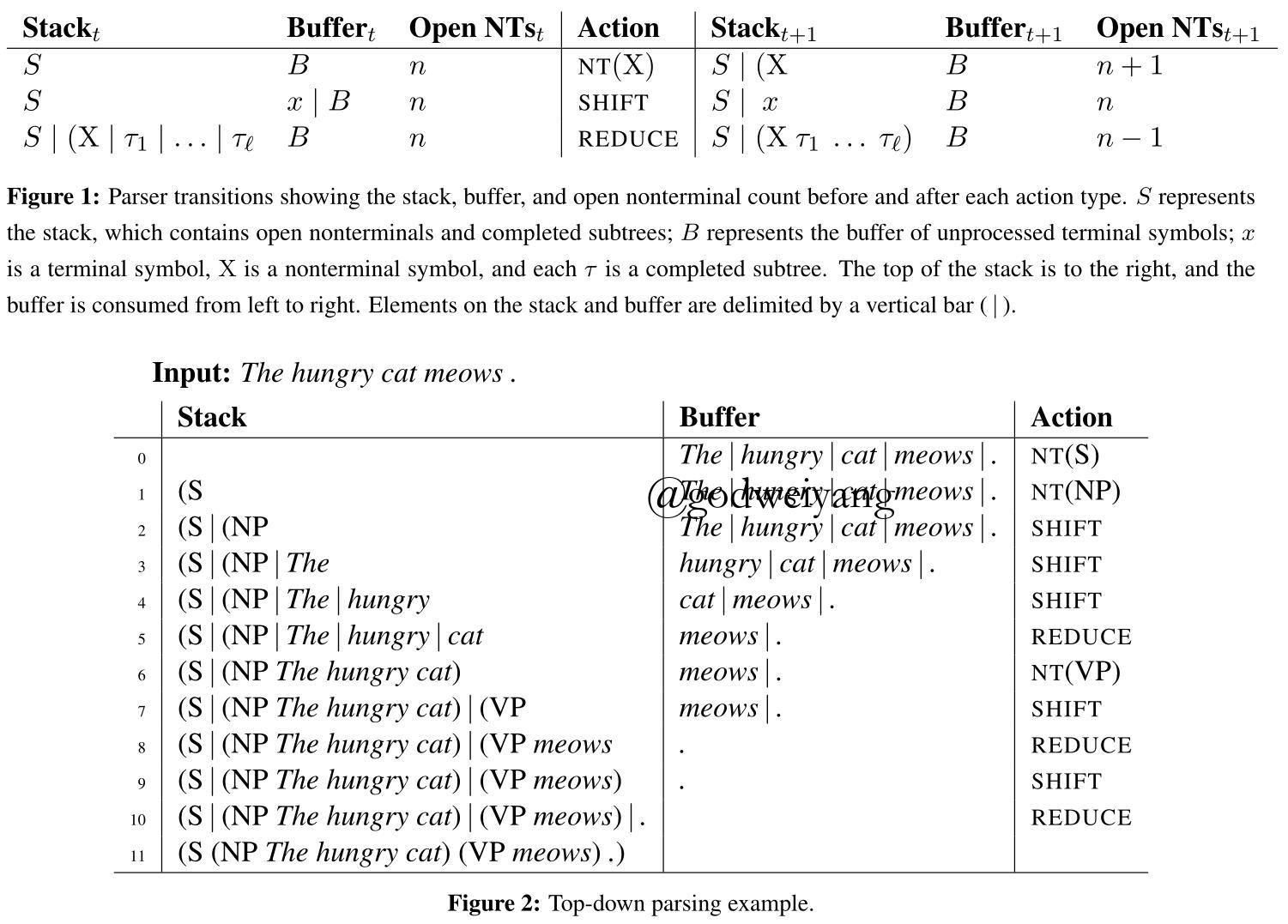• NT(X)动作只有当buffer不为空并且$n < 100$的时候才能进行。因为buffer空了的话就没有单词了，此时不可能移进新的非终结符了，并且要限制$n < 100$防止一元产生式无限生成下去。
• SHIFT动作只有当buffer不为空并且$n \ge 1$时才能进行。前者不用解释了，后者的话因为是top-down的，所以栈里至少要有一个父结点才能移进新的单词。
• REDUCE只有当栈顶不是没有归约的父结点才能进行。
• REDUCE只有当$n \ge 2$或者buffer为空时才能进行。这里要解释一下为什么$n \ge 2$，因为如果buffer不为空同时$n = 1$，那么这时候如果REDUCE的话，栈里就只剩一个非终结符了，只可能是根节点S，而buffer里还有单词，所以这是不可能的。

## 生成式系统

• 首先没有了输入的buffer，取而代之的是输出的buffer $T$。
• 其次因为没有输入单词了，所以在需要输入单词的时候采用GEN(x)动作来产生一个新的单词$x$，然后移进栈里，取代SHIFT动作。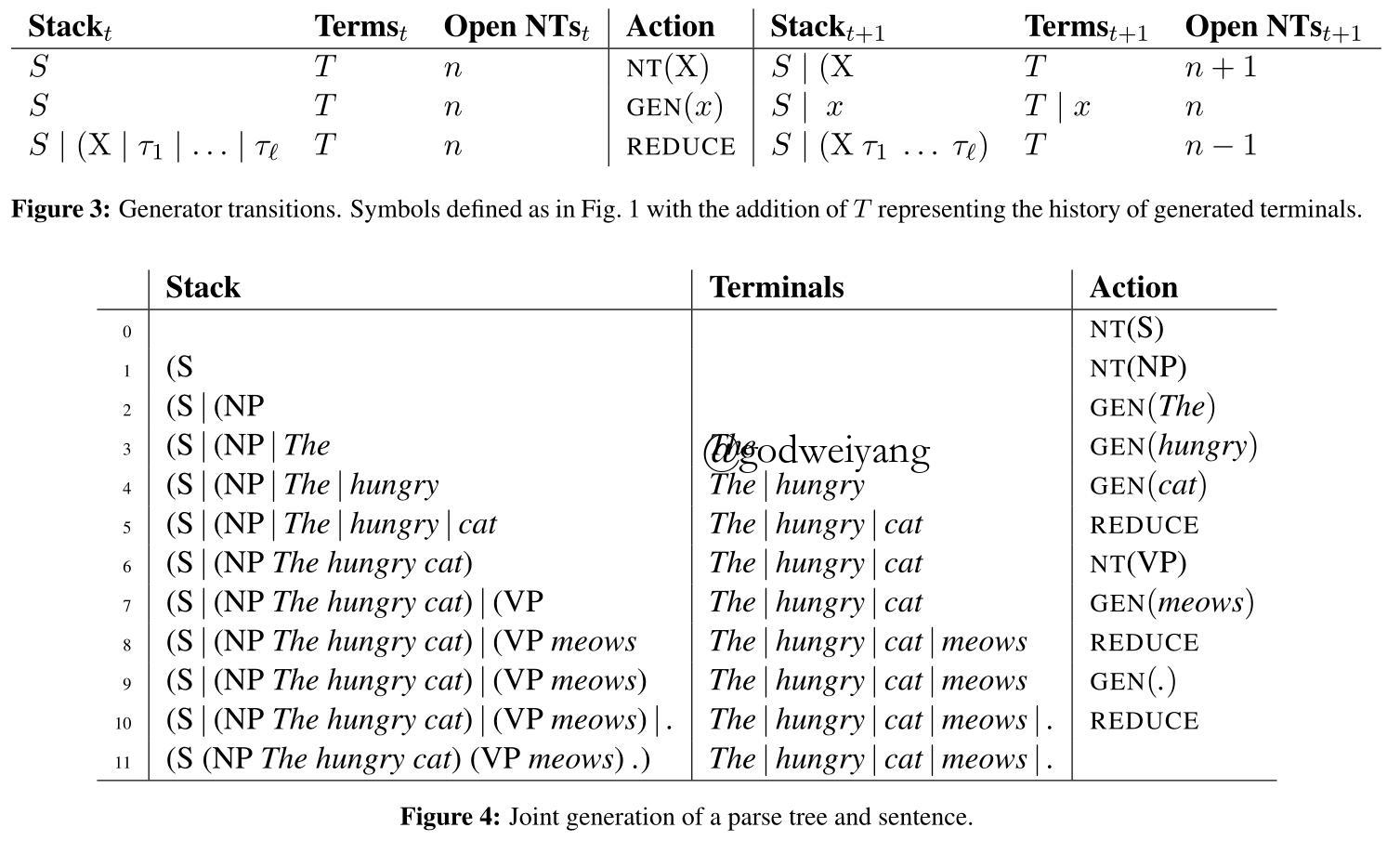• GEN(x)动作只有当$n \ge 1$时才能进行，上面SHIFT限制已经解释过了。
• REDUCE只有当$n \ge 1$或者buffer为空时才能进行。这里再次解释一下，上面判别式模型限制条件是$n \ge 2$，为什么这里就变成了$n \ge 1$？因为生成模型没有输入buffer，所以即使$n = 1$时REDUCE了，以后不要再GEN(x)即可，直接结束分析

# 生成式模型

$p(x,y) = \prod\limits_{t = 1}^{\left| {a(x,y)} \right|} {p({a_t}|{a_{ < t}})} = \prod\limits_{t = 1}^{\left| {a(x,y)} \right|} {\frac{ {\exp r_{ {a_t}}^T{u_t} + {b_{ {a_t}}}}}{ {\sum\nolimits_{a’ \in {\mathcal{A}_G}({T_t},{S_t},{n_t})} {\exp r_{a’}^T{u_t} + {b_{a’}}} }}}$

$u_t = \tanh (W[o_t; s_t; h_t] + c)$
$W$和$c$同样也包含在了RNNG参数集合$\Theta$里面，下图是三个LSTM的示例图：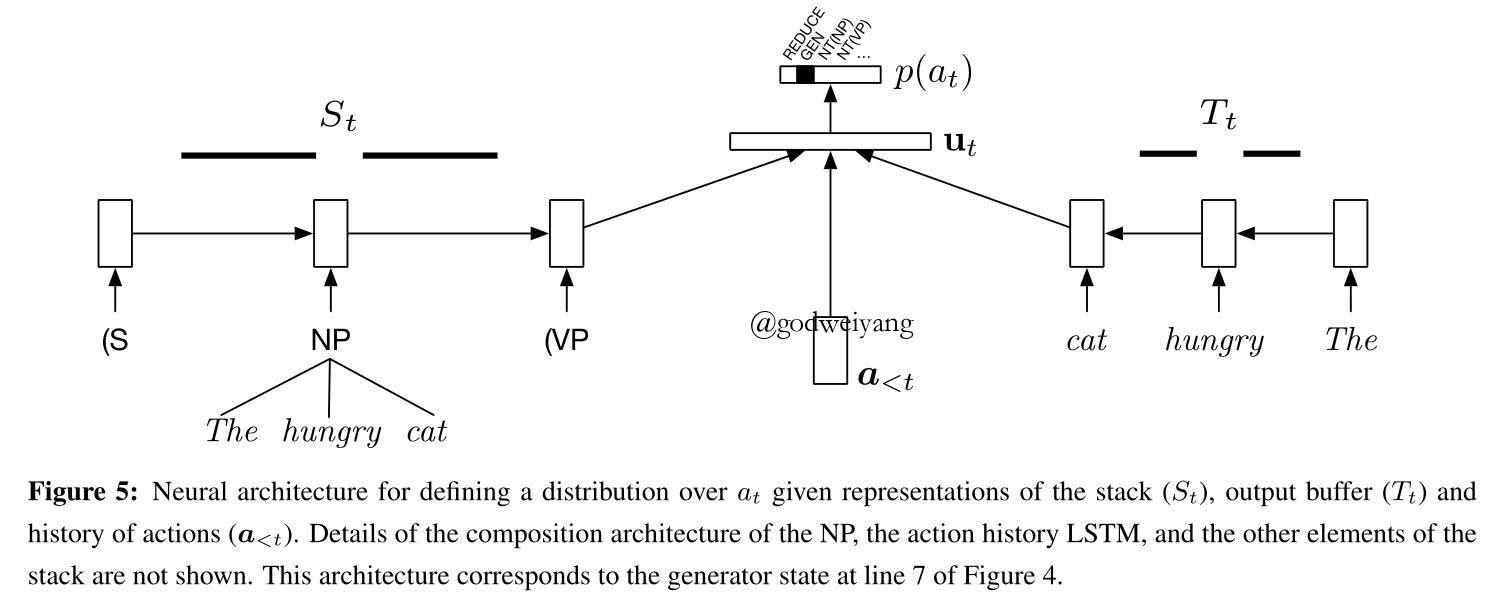## 句法成分组合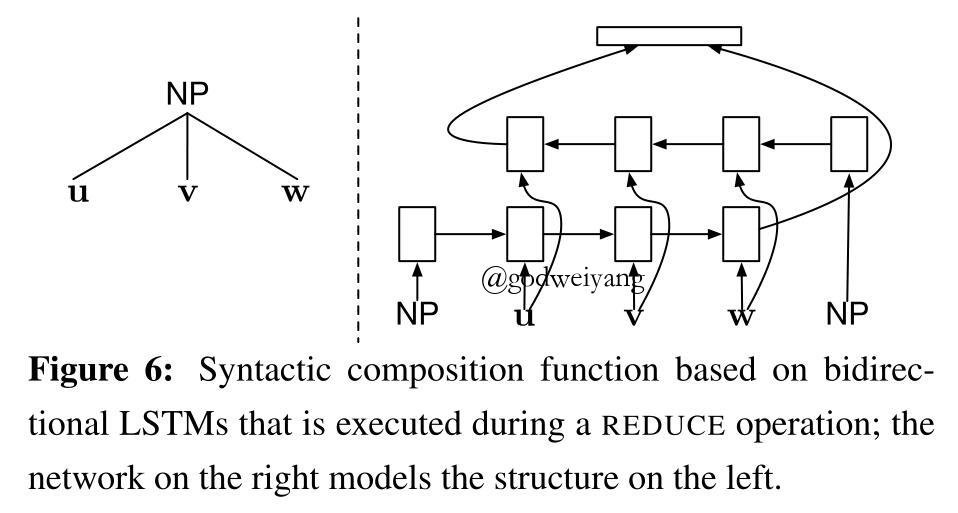# 通过重要性采样进行推理

$p(x) = \sum\nolimits_{y \in \mathcal{Y}(x)} {p(x,y)}$

• $p(y | x) > 0$可以推出$q(y | x) > 0$。
• 服从分布的样本很容易得到。
• $q(y | x)$可以直接计算得到。

$p(x) = \sum\nolimits_{y \in \mathcal{Y}(x)} {p(x,y)} = \sum\nolimits_{y \in \mathcal{Y}(x)} {q(y|x)w(x,y)} = {E_{q(y|x)}}w(x,y)$

${E_{q(y|x)}}w(x,y) \approx \frac{1}{N}\sum\limits_{i = 1}^N {w(x,{y_i})}$

# 实验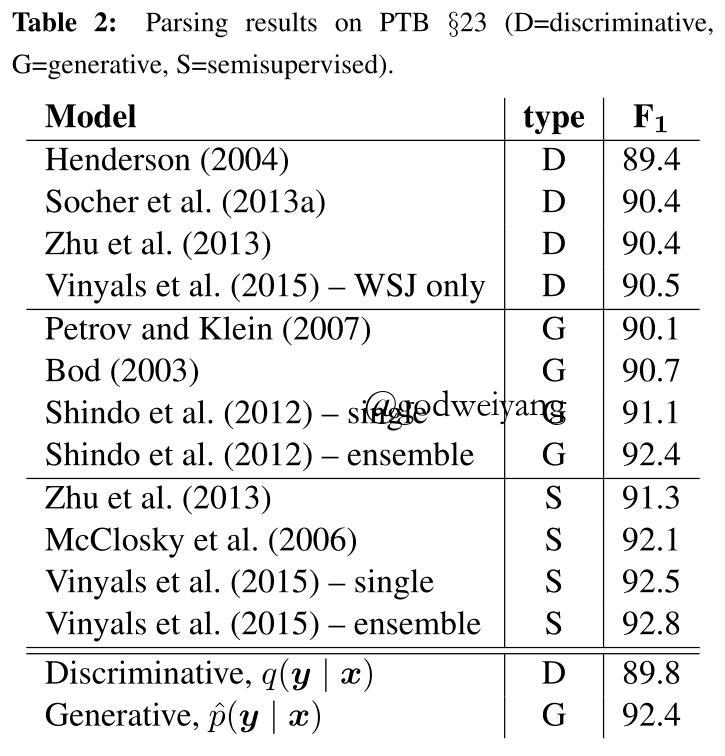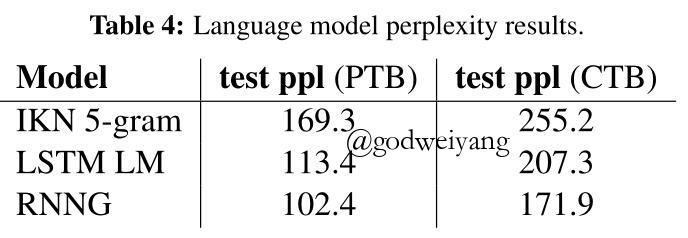# 总结

RNNG这个文法是个生成式模型，建模了句子和句法树的联合分布，稍稍修改即可应用到句法分析和语言模型中，效果也非常的好。

转载规则

《Recurrent Neural Network Grammars》 韦阳 采用 知识共享署名 4.0 国际许可协议 进行许可。
上一篇成分句法分析综述

2018-09-26In-Order Transition-based Constituent Parsing

2018-08-28
目录